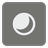Pearls in the Box
Simple
English DE FR RUTo start the game they put several black and white pearls in one of the boxes. Each robot has N moves, after which the initial set is being restored for the next game. Each turn, the robot takes a pearl out of the box and puts one of the opposite color back. The winner is the one who takes the white pearl on the Nth move.

Our robots don't like uncertainty, that's why they want to know the probability of drawing a white pearl on the Nth move. The probability is a value between 0 (0% chance or will not happen) and 1 (100% chance or will happen). The result is a float from 0 to 1 with two decimal digits of precision (±0.01).

You are given a start set of pearls as a string that contains "b" (black) and "w" (white) and the number of the move (N). The order of the pearls does not matter.Input: The start sequence of the pearls as a string and the move number as an integer.

Output: The probability for a white pearl as a float.

Example:

```checkio('bbw', 3) == 0.48
checkio('wwb', 3) == 0.52
checkio('www', 3) == 0.56
checkio('bbbb', 1) == 0
checkio('wwbb', 4) == 0.5
checkio('bwbwbwb', 5) == 0.48
```

How it is used: This task introduces you to the basics of probability theory and statistics. Fun fact: regardless of the initial state, as the number of moves increases, the probability approaches 0.5!

Precondition: 0 < N ≤ 20
0 < |pearls| ≤ 20